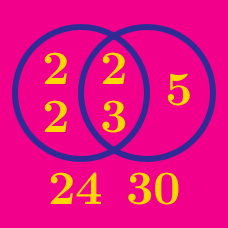Number Theory

# Relationship between GCD and LCM

What is the lowest common multiple of 7 and 11?

When the GCD of 2048 and 3072 is 1024, what is the LCM of 2048 and 3072?

$A$ and $B$ are positive integers such that

$A B=540, \quad \text{lcm}(A, B)=90.$

What is $\gcd(A, B)?$

If the GCD of $A$ and $B$ is $7,$ the LCM is $63,$ and $A>B,$ what is the value of $B?$

What is the lowest common multiple of 4 and 9?

×